# Excel VLOOKUP – Massive Guide with 8 Examples

VLOOKUP is one of the most useful and important functions in Microsoft Excel. It is generally used to look up a particular value in huge data sheets where manual intervention can be cumbersome. The alphabet ‘V’ in VLOOKUP stands for “Vertical” so this function is sometimes also called vertical lookup.

The term “Vertical” signifies that it can be used to look up values vertically i.e. it can be used to look up values inside a column.

You can also read my previous post: “How to alphabetize in Excel”, there I had used Excel VLOOKUP to sort a list.

Note: This is a long post of over 2700+ words. If you don’t want to read the whole post, you can use the below jump links to navigate to the desired section.

### Definition of VLOOKUP in Excel:

According to Microsoft Excel VLOOKUP can be defined as a function, “that looks for a value in the leftmost column of a table, and then returns a value in the same row from a column you specify. By default the table must be sorted in an ascending order.”

### What are the uses of Vertical Lookup Function?

This function is mostly used for following tasks:

1. To look up a single or a set of values from a data sheet.
2. To add a column to a datasheet from some other table, based on some unique (attribute)s.

### Syntax of VLOOKUP Function:

Its syntax is as follows:

`=VLOOKUP( lookup_value, table_array, column_index, range_lookup )`

Here, ‘`lookup_value`’ specifies the value to be searched inside the ‘`table_array`’. It can either be a value or a reference.

`table_array`’ is the range with two or more columns. ‘`table_array`’ argument can receive a range reference or a named range. The leftmost column of this range must contain the ‘`lookup_value`’.

`column_index`’ is the relative index of the column whose value needs to be returned by the VLOOKUP function. A ‘`column_index`’ 1 would return values from the first column in the ‘`table_array`’. Similarly ‘`column_index`’ 2 would return values from the second column in the ‘`table_array`’.

`range_lookup`’ is a Boolean value that specifies whether you want VLOOKUP to find an exact match or an approximate match. If its value is ‘True’ then either an approximate or an exact match will be returned. Here, if an exact match is not found, the next value that is less than ‘`lookup_value`’ is returned. If its value is ‘False’ then only exact match will be returned.

### Few Important points about Vertical Lookup function:

• VLOOKUP function performs a case-insensitive lookup.
• Excel VLOOKUP returns a ‘#N/A’ if it is not able to find the ‘`lookup_value`’ inside the ‘`table_array`’.
• It returns a ‘#VALUE!’ error if the value of ‘`column_index`’ is less than 1.
• It returns a ‘#REF!’ error if the value of ‘`column_index`’ is greater than the number of columns in the ‘`table_array`’.
• The default value of ‘`range_lookup`’ is TRUE. So, it is better to omit this argument in case you need to perform either an approximate or an exact match.
• Vertical Lookup allows you to use wildcard characters in the ‘`lookup_value`’ argument. We will discuss it later in this article.

## How to Use Excel VLOOKUP?

Before we understand how to use a Vertical Lookup function, we must understand what its objective is. Let’s try to understand this with a sample problem.

Suppose we have a table as shown below.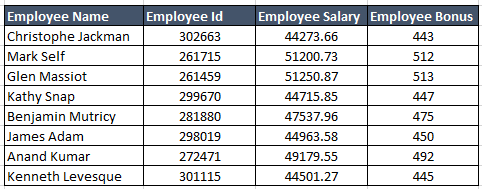Objective: Our objective is to find the salary of any particular employee (say: Benjamin Mutricy) based on his name.

Solution: Now, lets try to apply a VLOOKUP to find the solution.lookup_value: This is the value based on which the lookup is to be performed. In our case lookup_value is in the cell B4 i.e.  “Benjamin Mutricy”.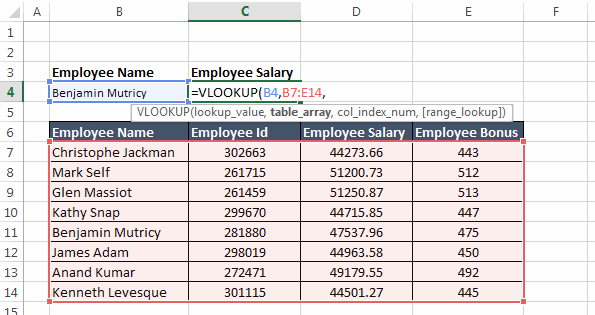table_array: This is the range of the table from which the values are to be fetched. Note that this ‘`table_array`’ should always contain ‘`lookup_value`’ in its leftmost column.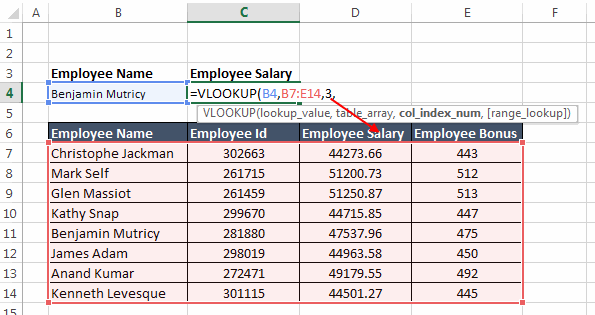col_index_num: This specifies the positional reference of the column that you want the VLOOKUP to return.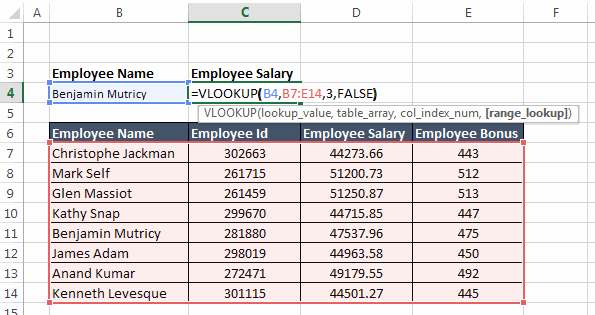range_lookup: This specifies that whether the match should be exact or approximate. FALSE specifies exact match.So, in this case the VLOOKUP function would be: `=VLOOKUP(B4,B7:D14,3,FALSE)`

### 5 Beginner level examples of VLOOKUP function:

Now let’s move to some examples:

Example 1: In this example we have a list of students with their scores. Now, here we need to find the score of a student with the First Name ‘Vincent’.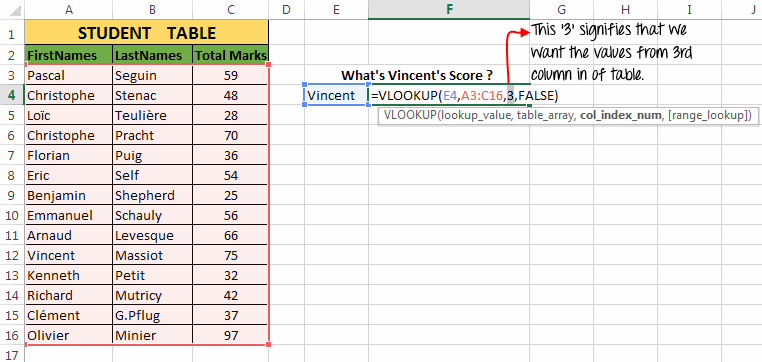To find the solution to our problem, we have used the vertical lookup as:

`=VLOOKUP(E4,A3:C16,3,FALSE)` and it gives the result 75.

Explanation:

• The first argument to the function i.e. ‘`lookup_value`’ = E4 (Reference of “Vincent”).
• Second argument i.e. ‘`table_array`’ = A3:C16 (Range of student table).
• Third argument i.e. ‘`column_index`’ = 3 (the column number whose value the VLOOKUP function should return).
• Fourth argument i.e. ‘`range_lookup`’ = FALSE (Signifies that we only want the exact match).

Example 2: In the next example we have the same table, but now our task is to find the last name of a student ‘Emmanuel’.So, we will use the VLOOKUP as: `=VLOOKUP(E4,A3:C16,2,FALSE)` and it results into “Schauly”.

Explanation:

• The first argument to the function i.e. ‘`lookup_value`’ = E4 (Reference of “Emmanuel”).
• Second argument i.e. ‘`table_array`’ = A3:C16 (Range of student table).
• Third argument i.e. ‘`column_index`’ = 2 (the column number whose value the VLOOKUP function should return).
• Fourth argument i.e. ‘`range_lookup`’ = FALSE (Signifies that we only want the exact match).

Example 3: In this example we have an “Element Table” table. The table contains 3 columns namely Atomic Mass, Density and Element Name.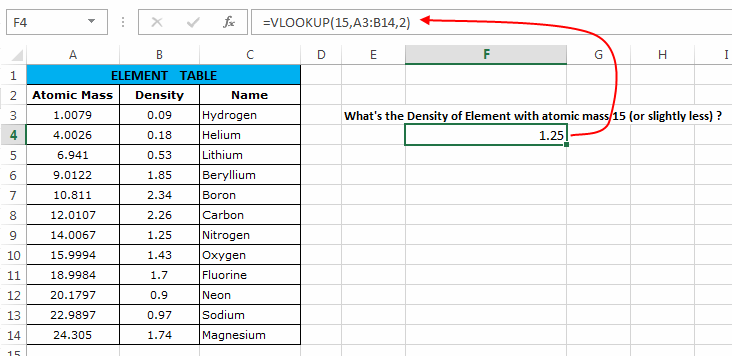Here we have to find the Density of an Element whose atomic mass is 15 or slightly less.

Now, here we can use the vertical lookup formula as: `=VLOOKUP(15,A3:B14,2)` which results into 1.25.

Explanation:

• The first argument to the function i.e. ‘`lookup_value`’ = 15 (Atomic Mass to be searched).
• Second argument i.e. ‘`table_array`’ = A3:B14 (Range of Element Table).
• Third argument i.e. ‘`column_index`’ = 2 (the column number whose value the vertical lookup function should return).
• Fourth argument i.e. ‘`range_lookup`’ is omitted and hence its value is true. So, first it searches 15 in the Atomic Mass column. And when it fails to find 15, it returns the density of element slightly less than 15.

Example 4: In this example we have 2 tables as shown below. Our task is to apply a Vertical Lookup based on ‘Element Names’ and fetch the melting points of the elements from the second table.This can be done by using the formula: `=VLOOKUP(C3,\$F\$3:\$G\$14,2,FALSE)`

After applying this formula for the first element we have to drag the formula below (using the fill handle) for other elements.

Explanation:

• The first argument to the function i.e. ‘`lookup_value`’ = C3 (Reference for first element).
• Second argument i.e. ‘`table_array`’ = \$F\$3:\$G\$14 (Range of Element Table 2) – If you are wondering what are these ‘\$’ signs along with the table reference, then you should read this post.
• Third argument i.e. ‘`column_index`’ = 2 (the column number whose value the vertical lookup function should return).
• Fourth argument i.e. ‘`range_lookup`’ = FALSE (Signifies that we only want the exact match).

Note that the values that I have explained above are only for the first element. Dragging this formula itself makes the necessary changes in the formula to make it correct for the below elements as well.

Example 5: In this example we will use wildcard operators along with Vertical Lookup. Here we have to find the score of the student whose first name starts with ‘A’.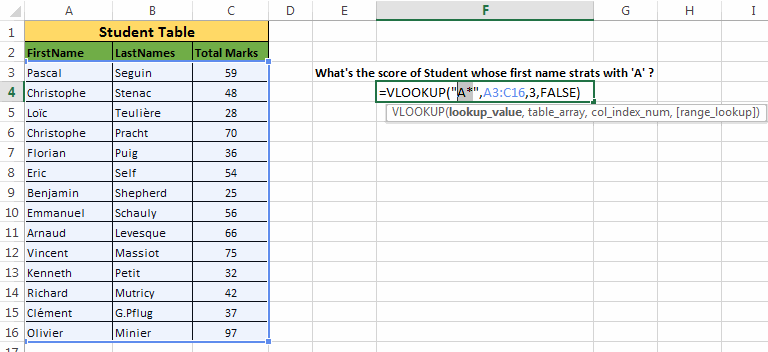For this we can use the formula: `=VLOOKUP("A*",A3:C16,3,FALSE)` which gives a result 66.

Explanation:

Generally, we can use following two wildcard operators with Excel VLOOKUP function.

WildcardDescription
‘?’Denotes any single character.
‘*’Denotes any number of characters

Note: Simply placing the tilde sign (~) before any wildcard character tells Excel that the wildcard character (‘*’ or ‘?’) should be treated as a string and not as wildcard operator.

• The first argument to the function i.e. ‘`lookup_value`’ = “A*” (Any word starting with ‘A’ alphabet).
• Second argument i.e. ‘`table_array`’ = A3:C16 (Range of Student Table).
• Third argument i.e. ‘`column_index`’ = 3 (the column number whose value the vertical lookup function should return).
• Fourth argument i.e. ‘`range_lookup`’ = FALSE (Signifies that we only want the exact match).

### Few Practical Examples of Vertical Lookup function:

Now let’s see some practical examples of VLOOKUP Function:

Example 6: Let’s say we have a list named “Child List” and another list with the name “Master list”. Now, using Vertical lookup we need to find if all the items in “Child List” are also present in the “Master list”.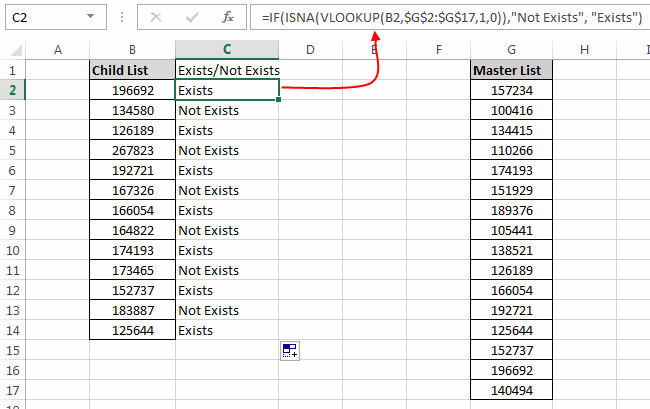In such a case we will use the formula: `=IF(ISNA(VLOOKUP(B2,\$G\$2:\$G\$17,1,0)),"Not Exists", "Exists")`

This formula uses If Statement, ISNA function and VLOOKUP.

Explanation:

Here, IF statement checks whether the output of VLOOKUP function is #NA Error or not. IF the output is #NA error, then it means that current child list item is not present in the master list. And hence the IF statement writes “Not Exists” in front of it. However if the item is present in the master list then it writes “Exists”.

Example 7: Translating scores to grades using vertical lookup. Let’s say we have a table with student scores and now we have to assign them a grade based on their scores.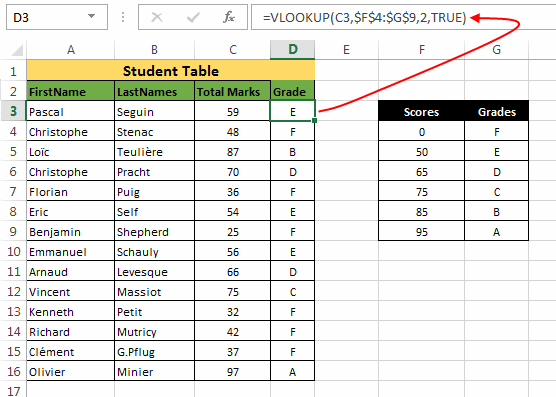For this we can use a VLOOKUP as: `=VLOOKUP(C3,\$F\$4:\$G\$9,2,TRUE)` and then drag this formula to below cells.

Explanation:

• The first argument to the function i.e. ‘`lookup_value`’ = C3 (Reference for first element).
• Second argument i.e. ‘`table_array`’ = \$F\$4:\$G\$9 (Range of scores and grades table).
• Third argument i.e. ‘`column_index`’ = 2 (the column number whose value the VLOOKUP function should return).
• Fourth argument i.e. ‘`range_lookup`’ = True (It matches both the exact values and values slightly lesser).

Example 8: Applying Vertical lookup using a table on another sheet or another workbook. In all the examples that we have discussed till now, we have applied VLOOKUP on tables that are on same worksheet. Now let’s redo the task in previous example but this time with the scores and grade table present on another worksheet sheet.In this example the “Student Table” is on ‘Sheet1’ while the “Grade and Score table” is on the ‘Sheet2’. So you can use a VLOOKUP as: `=VLOOKUP(C3,Sheet2!\$B\$3:\$C\$8,2,TRUE)`

Explanation:

• The first argument to the function i.e. ‘`lookup_value`’ = C3 (Reference for first element).
• Second argument i.e. ‘`table_array`’ = Sheet2!\$B\$3:\$C\$8 (Range of scores and grades table which is present on Sheet2).
• Third argument i.e. ‘`column_index`’ = 2 (the column number whose value the vertical lookup function should return).
• Fourth argument i.e. ‘`range_lookup`’ = True (It matches both exact and values slightly lesser).

Tip: While writing such VLOOKUP formulas you can simply navigate to the Sheet where the table is present and then select the required table. This will automatically insert the reference of that particular table in the formula.

See the below animation for more details:### How to Use VLOOKUP in VBA:

VLOOKUP function can also be used in VBA. Below is a sample code:

`Sub Vertical_lookup_test()Dim Result As VariantDim myVal As String ' Can be Integer, long, double etc.Dim Rng As RangeDim Clm As IntegerSet Rng = ActiveSheet.Range("A:E") ' Set RangemyVal = "Florian" ' Value to be searchedClm = 3 ' Column to be fetchedResult = Application.VLookup(myVal, Rng, Clm, False)If IsError(Result) Then Result = "Not found!"End IfMsgBox ResultEnd Sub`

Before using the above code make sure you change the following values:

1. Change `Set Rng = ActiveSheet.Range("A:E")` to the range that you wish to use.

2. Change `myVal = "Florian"` to the value that you want the vertical lookup to search. You can also use the InputBox function to get this value from user during the runtime.

3. Change `Clm = 3` to the column that you the VLOOKUP to fetch.

Recommended Reading : VLOOKUP In VBA – With Examples

### How to return multiple columns from a VLOOKUP?

Till now we have seen VLOOKUP functions that only returns a single column. But there are times when you need a VLOOKUP function to return multiple columns from the specified row.

So, you have to make a VLOOKUP formula that can fetch multiple columns. The basic idea behind this is, we will use VLOOKUP as an array function.

Follow the below steps to use this:

Suppose we need to fetch all the details of “Florian”. So we will proceed as:

1. Select the cells (cells equal to the number of columns that you wish to fetch) where you wish to populate the VLOOKUP results.

2. Next, without clicking anywhere else type the formula: `VLOOKUP("Florian",A:D,{1,2,3,4},FALSE)` in the Formula bar. The third argument i.e. {1,2,3,4} specifies the columns that need to be fetched.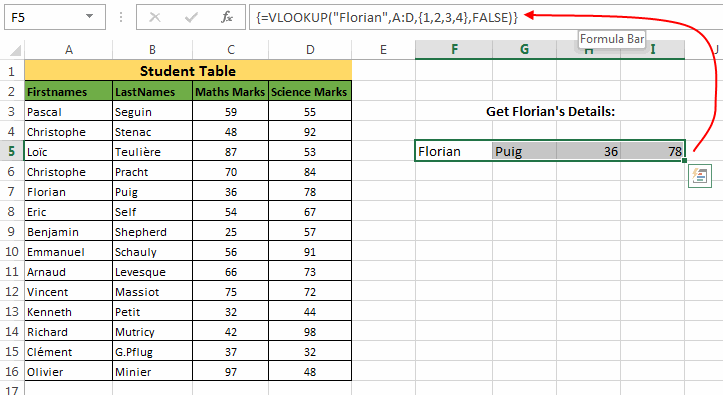3. After this simply hit the Ctrl + Shift + Enter keys. This will enclose the above formula in curly brackets and the cells that you had selected will show the fetched columns.

### Negative VLOOKUP:

In all the previous examples you must have seen that we have always used a ‘`lookup_value`’ that is present in the leftmost column of the range. So, the question arises, can we use a ‘`lookup_value`’ which is not in the leftmost column?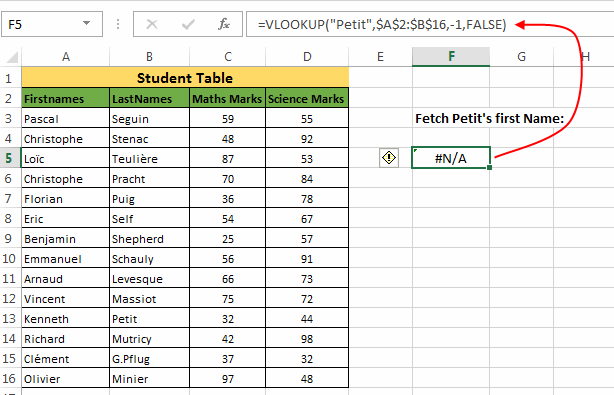See what happens when your ‘`lookup_value`’ is not on the leftmost side, and you enter a negative ‘`column_index`’ hoping to fetch the value to the left of ‘`lookup_value`’.

A simple way to do this is by building a user defined function (UDF). This function internally doesn’t use VLOOKUP but it can give you desired results. Below is the UDF:

`Function NEGATIVE_VLOOKUP(lookup_value, table_array As Range, col_index_num As Integer, CloseMatch As Boolean)Dim RowNbr As LongRowNbr = Application.WorksheetFunction.Match(lookup_value, table_array.Resize(, 1), CloseMatch)NEGATIVE_VLOOKUP = table_array(RowNbr, 1).Offset(0, col_index_num)End Function`

This UDF uses Match and Offset functions to accomplish the task.

How to use this Negative VLOOKUP UDF:

Let’s understand how to use this function. In our example we have a Student Table and we have to find the first name of “Petit”.

So, we will use this formula as: `=NEGATIVE_VLOOKUP("Petit",B:B,-1,FALSE)`Explanation:

• The first argument to this UDF is the ‘`lookup_value`’.
• Second argument is the range where this UDF should search for ‘`lookup_value`’.
• Third argument is the positional reference of the column which this UDF should return.
• Last argument is the Boolean value specifying exact or approximate match.

Similarly, in the same table if we have to find the first name of the student with 98 Marks in Science, then the formula would be:

`=NEGATIVE_VLOOKUP(98,D:D,-3,FALSE)`

### Create a multiple criteria VLOOKUP:

While doing data analysis in excel sometimes you may run into situations where you need to apply a VLOOKUP based on two keys (i.e. based on two ‘`lookup_values`’).

For example: We have a Student table as shown in the below image. Now, as you can see that there are two students with First Name “Christophe” (at A4 and A6). So, applying a VLOOKUP on first name can cause inconsistency. Hence we will apply a VLOOKUP based on both First name as well as the Last name.

But as we know that Excel VLOOKUP can only have a single ‘`lookup_value`’. So we need to create a helper column by appending first name and last name. We can do this by writing. `=A3&" "&B3`Now, we have combined two keys together and hence this new key column would contain unique values.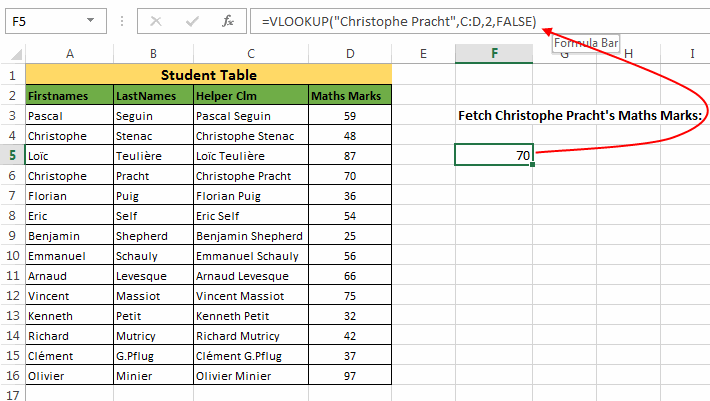Next, we can simply apply a Vertical lookup as: `=VLOOKUP("Christophe Pracht",C:D,2,FALSE)`

So, this was all about Excel VLOOKUP. Do read this post if this long article has bored you and don’t forget to share your ideas and thoughts with us in the comments section.About Ankit Kaul

Ankit is the founder of Excel Trick. He is tech Geek who loves to sit in front of his square headed girlfriend (his PC) all day long. :D. Ankit has a strong passion for learning Microsoft Excel. His only aim is to turn you guys into 'Excel Geeks'.

1.amitsays

Thanks Again.

•Ankit Kaulsays

You’re Welcome, Amit. 🙂

2.Ahmed Al Badrisays

Thank you Ankit. The tutorial is awesome, helpful and detailed…
Earlier, I had read multiple tutorials in the subject. But couldn’t get anything.
Your article is simply great… While reading I have tried a couple of things…
And can say I am now able to understand VLOOKUP now.
This is the best training session.

Thanks a lot… 🙂

•Ankit Kaulsays

Subscribe to the updates and stay tuned for more.

3.Jamiesays

Hi! This is very helpful. But may I ask one more thing.
I can’t figure out? What if there are 5 Christophe’s on the array. And they all have different surnames.
With different maths marks of course.
So, in such a scenario. How can I fetch math marks for all of them.
Please assume that: In the data array that I’m using. There are multiple instances rows. These rows are duplicate. I’m working on 35,000 rows. With duplicates. Sorry. 🙂 I badly need some help. Thanks!

•Ankit Kaulsays

Hi Jamie,
In your case you could use a formula by combining Index and Match functions.
Oscar has explained it in a very nice way here.
Hope it helps. 🙂

4.Lucasays

I have a problem in using vlookup.
I need to summarize the value of the column.
After performing a vlookup match.
Example: Col are as follows- Product, Brand, Salesman.
Now if I want to get the summary of sales done by a salesman LMN using Vlookup. Can this be done?

•Ankit Kaulsays

Hi Luca.
You can use sumif formula for this.
Visit this post here.
And feel free to ask if you need any help 🙂

5.Adisays

Great post. But I have a question.
Can I use Vlooup to return cell data and comments.
I want to apply a vlookup. And get the values and comments.
Is this possible?

•Ankit Kaulsays

Adi, Vlookup is not designed for such things.
But you can write a UDF for this.
Let me know if you need any help.
I will be more than happy to help.
Thanks 🙂

6.Jericcosays

Nice post. But I wanted to ask one thing.
How to apply lookup from a different sheet.
For example: I mean, I have one set of values on one sheet. And some other values on a different sheet.
So, how can I achieve a vlookup between them.

•Ankit Kaulsays

Hi Jerico,

You can easily do this. I have explained this in Example 8.
Let me know if you still need some help. 🙂

7.Paulsays

Ankit, nice writeup.
•Ankit Kaulsays